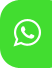## Product Detail## Ignou Solved Assignment for ECO-07: Element of Statistics

University  IGNOU
Service Type Solved Assignment
Course B.Com
Semester
Short Name or Subject Code ECO-07: Element of Statistics
Product B.Com of Solved Assignment (IGNOU)
Pattern
Price
Click to view price

Attempt all the questions

1. What is statistical survey? Discuss the steps to be followed while conducting statistical survey. (20)

2. Central tendency, dispersion an skewness are three different measures to analyse numerical data. Comment. (20)

3. Calculate a) Mean, b) Variance and c) Standard deviation from the following frequency distribution:-

Classes                    Frequency

1-10                              11

10-20                             29

20-30                             18

30-40                               4

40-50                              5

50-60                               3

4. a) What is the relationship between Mean. Median and Mode:

(i) Symmetrical curve

(ii) A negatively slewed curve

(iii) A positively skewed curve b) For a frequency distribution: Q3-Q2 = 40 & Q2-Q1=60 Find coefficient of skewness. (10+10)

5. Write short notes on the following - (5×4)

a) Principle of preparing graphs.

b) Statistical Table

c) Data Array

d) Properties of Normal Curve.